# Assignments

Logarithms are widely used in mathematics and in application areas such as economics and engineering. Therefore it is important to gain the necessary skills in working with logarithms. The following assignments are examples.

1. Calculate: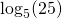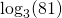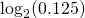Solution

2. Calculate: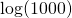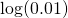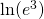Solution

3. Sketch the graphs of: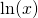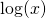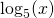Solution

4. Under which conditions is the following logarithmic function defined?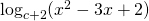Solution

5. Sketch the graph of the following logarithmic function: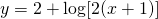Solution

6. Using their graphs shows that the following functions (a logarithmic and an exponential function):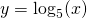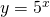are inverse functions. Which line is the symmetry axis?

Solution

7. Draw the graph of the function: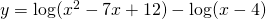Solution

8. Show the following relation: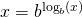Solution

9. Solve: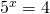Solution

10. Solve: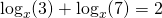Solution

0